• 2021-03-13 16:09:22

在之前的学习中，主要基于充分统计量给出点估计，并且注重于点估计的无偏性与相合性。然而，仅有这两个性质是不足的，无偏性只能保证统计量的均值与待估参数一致，却无法控制统计量可能偏离待估参数的程度；相合性只能在大样本下保证统计量到均值的收敛性，但却对小样本情形束手无策。今天我们将注重于统计量的有效性，即无偏统计量的抽样分布的方差。由于本系列为我独自完成的，缺少审阅，如果有任何错误，欢迎在评论区中指出，谢谢！

目录

Part 1：一致最小方差无偏估计

首先考虑这样的问题：如何刻画一个统计量的有效程度？注意到，一个统计量的取值既可能高于待估参数，亦可能低于待估参数，要综合考虑统计量对待估参数误差，需要用平方均衡这种双向偏差，因此，提出均方误差的概念：若$$\hat g(\boldsymbol{X})$$是$$g(\theta)$$的估计量，则$$\hat g(\boldsymbol{X})$$的均方误差定义为

$\mathrm{MSE}(\hat g(\boldsymbol{X}))= \mathbb{E}[\hat g(\boldsymbol{X})-g(\theta)]^2.$

对于确定的统计量$$\hat g(\boldsymbol{X})$$而言，$$\mathrm{MSE}(\hat g(\boldsymbol{X}))$$是$$\theta$$的函数。显然，一个统计量的均方误差越小，它就越在待估参数真值附近环绕，由此，用统计量的一次观测值作为待估参数的估计就有着越大的把握。

如果对于$$g(\theta)$$的两个估计量$$\hat g_1(\boldsymbol{X})$$和$$\hat g_2(\boldsymbol{X})$$，恒有$$\mathrm{MSE}(\hat g_1(\boldsymbol{X}))\le \mathrm{MSE}(\hat g_2(\boldsymbol{X}))$$，且严格不等号至少在某个$$\theta$$处成立，就称$$\hat g_1(\boldsymbol{X})$$在均方误差准则下优于$$\hat g_2(\boldsymbol{X})$$。如果我们能找到均方误差最小的统计量$$\hat g(\boldsymbol{X})$$，就相当于找到了均方误差准则下的最优统计量。

不过，均方误差是$$\theta$$的函数，这就导致了某些统计量在$$\theta=\theta_1$$时均方误差小，在$$\theta=\theta_2$$时均方误差大，一致最小均方误差估计量便不存在，需要增加约束条件，找到更可能存在的“最优”。

基于此，我们提出一致最小方差无偏估计(UMVUE)的概念，它将$$g(\theta)$$的估计量限制在了无偏估计之中，这使得UMVUE的存在可能性得以提高。并且，由于$$\mathbb{E}(\hat g(\boldsymbol{X}))=g(\theta)$$，所以

$\mathrm{MSE}(\hat g(\boldsymbol{X}))=\mathbb{E}(\hat g(\boldsymbol{X})-g(\theta))^2=\mathbb{E}[\hat g(\boldsymbol{X})-\mathbb{E}(\hat g(\boldsymbol{X}))]^2=\mathbb{D}(\hat g(\boldsymbol{X})),$

即无偏估计的均方误差就是无偏估计的方差。

不过首先要提出的是，UMVUE往往比一致最小均方误差估计量更容易存在，但依然不是所有参数都存在UMVUE的，并且，甚至可能有的参数根本不存在无偏估计。

以下是一个典型的不存在无偏估计的例子：$$X\sim B(n,p)$$，参数$$g(p)=1/p$$的无偏估计不存在。书上给出的证明过程如下：

首先，无偏估计不依赖于样本容量，故假设$$n=1$$。若$$g(p)$$有无偏估计$$\hat g(X)$$，则由于$$X=0,1,\cdots,n$$，故$$\hat g(X)$$的取值只可能是$$a_0,a_1,\cdots,a_n$$，可以写出其期望为

$\mathbb{E}(\hat g(X))=\sum_{j=0}^n a_jC_n^jp^j(1-p)^{n-j},$

当$$\hat g(X)$$为无偏估计时，成立以下等式：

$\sum_{j=0}^na_jC_n^jp^j(1-p)^{n-j}=\frac{1}{p},$

即对于某个多项式$$f\in\mathcal P_{n+1}(\mathbb{R})$$，有

$f(p)=0,\quad \forall p\in(0,1).$

显然，要使上式恒成立，除非$$f$$为零多项式。但$$f$$并不是零多项式，这意味着$$f(p)=0$$只会在至多$$n+1$$个点处成立，这与无偏性要求矛盾。因此，$$g(p)=1/p$$不存在无偏估计。

我们将存在无偏估计的待估参数称为可估参数，因此UMVUE仅对可估参数作讨论。我们的任务，就是用一定的方法，找到可估参数的UMVUE。

Part 2：改进无偏估计量

无偏估计量有许多，比如正态分布$$N(\mu,\sigma^2)$$中，$$\mu$$的无偏估计就有$$X_1,2X_2-X_1,\bar X$$等。充分统计量的条件期望法是改进无偏估计量的一个典型方式，它基于如下的定理：设$$T=T(\boldsymbol{X})$$是一个充分统计量，$$\hat g(\boldsymbol{X})$$是$$g(\theta)$$的一个普通无偏估计量，则$$h(T)=\mathbb{E}[\hat g(\boldsymbol{X})|T]$$是$$g(\theta)$$的无偏估计，且

$\mathbb{D}(h(T))\le \mathbb{D}(\hat g(\boldsymbol{X})),\quad \forall \theta\in\Theta.$

等号成立当且仅当$$\hat g(\boldsymbol{X})=h(T)$$是均方条件下成立的。它的证明不是很有必要掌握，权当了解。

因为$$T$$是$$g(\theta)$$的充分统计量，故$$\mathbb{E}(\hat g(\boldsymbol{X})|T)$$与待估参数$$g(\theta)$$无关，可以作为统计量，即

$h(T)=\mathbb{E}(\hat g(\boldsymbol{X})|T)$

是合理定义的统计量。下证其无偏性，由全期望公式，有

$\mathbb{E}(h(T))=\mathbb{E}[\mathbb{E}(\hat g(\boldsymbol{X})|T)]=\mathbb{E}(\hat g(\boldsymbol{X}))=g(\theta).$

最后证明其比$$\hat g(\boldsymbol{X})$$更有效，利用一个常用的拆分技巧，得到

\begin{aligned} \mathbb{D}(\hat g(\boldsymbol{X}))&=\mathbb{D}[\hat g(\boldsymbol{X})-h(T)+h(T)]\\ &=\mathbb{D}(h(T))+\mathbb{D}(\hat g(\boldsymbol{X})-h(T))+2\mathrm{Cov}(h(T),\hat g(\boldsymbol{X})-h(T)), \end{aligned}

然后证明交叉项为0，这里需要再次用到全期望公式，有

\begin{aligned} &\quad \mathrm{Cov}(h(T),\hat g(\boldsymbol{X})-h(T))\\ &=\mathbb{E}[h(T)-g(\theta)][\hat g(\boldsymbol{X})-h(T)]\\ &=\mathbb{E}[\mathbb{E}[(h(T)-g(\theta))(\hat g(\boldsymbol{X})-h(T))|T]]\\ &=\mathbb{E}[(h(T)-g(\theta))(\mathbb{E}(\hat g(\boldsymbol{X})|T)-h(T)]\\ &=0. \end{aligned}

最后的等号是因为$$h(T)=\mathbb{E}(\hat g(\boldsymbol{X})|T)$$，于是代回就得到

$\mathbb{D}(\hat g(\boldsymbol{X}))=\mathbb{D}(h(T))+\mathbb{D}(\hat g(\boldsymbol{X})-h(T))\ge \mathbb{D}(h(T)).$

等号成立当且仅当$$\mathbb{D}(\hat g(\boldsymbol{X})-h(T))^2=\mathbb{E}(\hat g(\boldsymbol{X})-h(T))^2=0$$。

这个定理的重要意义在于，如果给定的无偏估计量不是充分统计量的函数，则可以通过条件期望法，将其转变成一个充分统计量的函数作为新的统计量，并且新的统计量总是更有效的。此外，这也对我们寻找UMVUE提出启示：UMVUE一定是充分统计量的函数。如果不然，则可以通过对充分统计量求期望，得到一个更有效的统计量。

不过，改进后的充分统计量函数，尽管是更为有效的参数估计，但却并不一定是UMVUE，下面的定理将给出一个验证点估计是否为UMVUE的方法。

Part 3：零无偏估计法

零无偏估计法是用于判断某个估计量是否为UMVUE的方法，为此，首先要提出什么是零无偏估计。顾名思义，零无偏估计即零的无偏估计量，对某个统计量$$l(\boldsymbol{X})$$，如果有$$\mathbb{E}(l(\boldsymbol{X}))=0$$，则称$$l(\boldsymbol{X})$$是一个零无偏估计量；如果统计量$$T$$是待估参数$$g(\theta)$$的充分统计量，且$$\mathbb{E}(h(T))=0$$，则$$h(T)$$也称为$$g(\theta)$$的零无偏估计量。

零无偏估计法的思想、证明过程都与上述的充分统计量条件期望法类似。如果$$\hat g(\boldsymbol{X})$$是UMVUE，则对于任意其他无偏估计$$\hat g_1(\boldsymbol{X})$$，都可以视为$$\hat g_1(\boldsymbol{X})=\hat g(\boldsymbol{X})+l(\boldsymbol{X})$$，显然这里$$\mathbb{E}(l(\boldsymbol{X}))=0$$，要使$$\hat g_1(\boldsymbol{X})$$的方差大于$$\hat g(\boldsymbol{X})$$，可以进行拆分，即

$\mathbb{D}(\hat g_1(\boldsymbol{X}))=\mathbb{D}(\hat g(\boldsymbol{X}))+\mathbb{D}(l(\boldsymbol{X}))+2\mathrm{Cov}(\hat g(\boldsymbol{X}),l(\boldsymbol{X})).$

如果最后的协方差项为0，则必有$$\mathbb{D}(\hat g_1(\boldsymbol{X}))\ge \mathbb{D}(\hat g(\boldsymbol{X}))$$。综合以上讨论，给出零无偏估计法验证UMVUE的方式。

设$$\hat g(\boldsymbol{X})$$是$$g(\theta)$$的一个无偏估计，$$\mathbb{D}(\hat g(\boldsymbol{X})) $\mathrm{Cov}(\hat g(\boldsymbol{X}),l(\boldsymbol{X}))=0,$ 则\(\hat g(\boldsymbol{X})$$是$$g(\theta)$$的UMVUE。

如果$$\hat g(\boldsymbol{X})$$满足与任何零无偏估计无关，则它是UMVUE，这是一个充分条件。但反之，它也是一个必要条件，即UMVUE必定与任何零无偏估计量无关。

如果不然，设$$\mathrm{Cov}(\hat g(\boldsymbol{X}),l(\boldsymbol{X}))=b(\theta)\ne 0$$，则可以假设$$\mathbb{D}(l(\boldsymbol{X}))=a^2(\theta)>0$$。现在固定$$\theta=\theta_0$$为常数，并设$$a(\theta_0)=a,b(\theta_0)=b$$，只要

$\mathbb{D}(l(\boldsymbol{X}))+2\mathrm{Cov}(\hat g(\boldsymbol{X}),l(\boldsymbol{X}))=a^2(\theta_0)+2b(\theta_0)=a^2+2b<0,$

就能找到一个在$$\theta=\theta_0$$处，比$$\hat g(\boldsymbol{X})$$方差更小的无偏估计，那么$$\hat g(\boldsymbol{X})$$就不是UMVUE。注意到，如果$$l(\boldsymbol{X})$$是零无偏估计，则$$\forall k\ne 0$$，$$kl(\boldsymbol{X})$$也是零无偏估计，就有

$\mathbb{D}(kl(\boldsymbol{X}))+2\mathrm{Cov}(\hat g(\boldsymbol{X}),kl(\boldsymbol{X}))=k^2a^2+2bk,$

取$$k$$值为

$\left\{\begin{array}l -\frac{2b}{a}0; \\ 0 就使得$$k^2a^2+2bk<0$$成立，于是 \[\hat g_1(\boldsymbol{X})\xlongequal{def}\hat g(\boldsymbol{X})+kl(\boldsymbol{X})$

是$$g(\theta)$$的无偏估计量，且当$$\theta=\theta_0$$时$$\mathbb{D}(\hat g_1(\boldsymbol{X})) 有了这个方法，我们可以验证一些常用的充分统计量是UMVUE了。现以正态分布\(N(\mu,\sigma^2)$$的充分统计量$$(\bar X,S^2)$$为例，它们是否是UMVUE呢？如果直接验证会稍显繁琐，对零无偏估计法稍加修改可以得到以下的推论：

如果$$T$$是充分统计量且$$h(T)$$是$$g(\theta)$$的一个无偏估计，对任何$$\theta\in\Theta$$与一切零无偏估计量$$\delta (T)$$都有

$\mathrm{Cov}(h(T),\delta(T))=\mathbb{E}(h(T)\delta(T))=0,$

则$$h(T)$$是UMVUE。

这里只是将样本的函数改成了充分统计量的函数，以上证明过程是依然适用的。并且，由于UMVUE一定是充分统计量的函数，因此这个推论会更有应用意义。

对于正态分布而言，充分统计量可以视为

$T_1=\frac{1}{n}\sum_{j=1}^n X_j,\quad T_2=\sum_{j=1}^n (X_j-\bar X)^2,\\ T_1\sim N\left(\mu,\frac{\sigma^2}{n}\right),\quad \frac{T_2}{\sigma^2}\sim\chi^2(n-1)\Rightarrow T_2\sim\Gamma\left(\frac{n-1}{2},\frac{1}{2\sigma^2} \right).$

由于$$T_1,T_2$$独立，所以其联合密度函数容易写出，有

$f_1(t_1)=\frac{\sqrt{n}}{\sqrt{2\pi \sigma^2}}\exp\left\{-\frac{n(t_1-\mu)^2}{2\sigma^2} \right\},\\ f_2(t_2)=\frac{1}{2^{\frac{n-1}{2}}\Gamma(\frac{n-1}{2})\sigma^{n-1}}t_2^{\frac{n-1}{2}-1}e^{-\frac{t_2}{2\sigma^2}},\\ f(t_1,t_2)=\frac{C}{\sigma^n}t_2^{\frac{n-1}{2}-1}\exp\left\{-\frac{n(t_1-\mu)^2+t_2}{2\sigma^2} \right\}.$

如果$$\delta(t_1,t_2)$$是零均值的，则有

$\mathbb{E}(\delta(t_1,t_2))=\frac{C}{\sigma^n}\int_{-\infty}^\infty \delta(t_1,t_2)\cdot t_2^{\frac{n-1}{2}-1}\exp\left\{-\frac{n(t_1-\mu)^2+t_2}{2\sigma^2} \right\}\mathrm{d}t_1\mathrm{d}t_2=0.$

令$$\exp$$部分为$$H(\mu,\sigma^2)$$，也就是我们获得了这个关键的等式(它是证明的核心)：

$\int_{-\infty}^\infty\delta(t_1,t_2)\cdot t_2^{\frac{n-1}{2}-1}H(\mu,\sigma^2)\mathrm{d}t_1\mathrm{d}t_2=0$

要验证$$\mathbb{E}(t_1,\delta(t_1,t_2))$$与$$\mathbb{E}(t_2,\delta(t_1,t_2))$$是否为0，先从第一个入手，写出其表达式为

$\mathbb{E}(t_1,\delta(t_1,t_2))=\frac{C}{\sigma^n}\int_{-\infty}^{\infty}\delta(t_1,t_2)t_1t_2^{\frac{n-1}{2}-1}H(\mu,\sigma^2)\mathrm{d}t_1\mathrm{d}t_2.$

要验证$$\mathbb{E}(t_1,\delta(t_1,t_2))=0$$，实际上就是验证

$\int_{-\infty}^\infty \delta(t_1,t_2)t_1t_2^{\frac{n-1}{2}-1}H(\mu,\sigma^2)\mathrm{d}t_1\mathrm{d}t_2\stackrel{?}=0.$

不要被这个庞然大物吓到，事实上我们唯一的条件只有$$\mathbb{E}(\delta(t_1,t_2))=0$$所对应的等式，注意到上面的等式无论$$\mu,\sigma$$的真值是多少都应该成立，所以是$$\mu,\sigma$$的二元函数，我们能做的事也很有限——对参数求导。这里涉及到了求导与积分是否可交换的问题，我们姑且不考虑，默认视为可交换即可，由于$$\sigma^2$$很复杂，所以对$$\mu$$求导即可。唯一含有$$\mu$$的项是积分号中间的$$\exp$$部分，其导数为

$H(\mu,\sigma^2)=\exp\left\{-\frac{n(t_1-\mu)^2+t_2}{2\sigma^2} \right\},\\ \frac{\partial H(\mu,\sigma^2)}{\partial \mu}=\frac{2n(t_1-\mu)}{\sigma^2}H(\mu,\sigma^2),$

于是就有

$\frac{\partial \mathbb{E}(\delta_1,\delta_2)}{\partial\mu}=\frac{C}{\sigma^n}\int_{-\infty}^\infty\delta(t_1,t_2)t_2^{\frac{n-1}{2}-1}\frac{2n(t_1-\mu)}{\sigma^2}H(\mu,\sigma^2)\mathrm{d}t_1\mathrm{d}t_2=0,$

将求导后得到的式子展开，提取出参数并代入上面的结果，就有

$\int_{-\infty}^\infty\delta(t_1,t_2)t_1t_2^{\frac{n-1}{2}-1}H(\mu,\sigma)\mathrm{d}t_1\mathrm{d}t_2=0,$

也就说明了$$\mathbb{E}(t_1,\delta(t_1,t_2))=0$$，由$$\delta(t_1,t_2)$$的任意性以及$$T_1$$的无偏性，可知$$\bar X$$是$$\mu$$的UMVUE。

下一步证明$$T_2/(n-1)$$是$$\sigma^2$$的UMVUE，也就是证明$$\mathbb{E}(t_2,\delta(t_1,t_2))=0$$，同样写出需要验证的等式为

$\int_{-\infty}^\infty\delta(t_1,t_2) t_2^{\frac{n-1}{2}}H(\mu,\sigma^2)\mathrm{d}t_1\mathrm{d}t_2\stackrel{?}=0.$

现在我们尝试将关键的等式对$$\sigma^2$$求导，同样，先计算$$H$$对$$\sigma^2$$的偏导，有

$H(\mu,\sigma^2)=\exp\left\{-\frac{n(t_1-\mu)^2+t_2}{2\sigma^2} \right\},\\ \frac{\partial H(\mu,\sigma^2)}{\partial \sigma^2}=\frac{n(t_1-\mu)^2+t_2}{2\sigma^4}H(\mu,\sigma^2),$

可以看到，这里除了出现已经确认代入能为0的常数项和$$t_1$$外，还多了$$t_1^2$$与$$t_2$$的项，$$t_2$$就是我们的目标，所以再处理一下$$t_1^2$$这一项。显然，对$$\mu$$求导一次能得到$$t_1$$的一次项，那么对$$\mu$$求二阶导，就能得到$$t_1^2$$项，所以

$\frac{\partial H(\mu,\sigma^2)}{\partial \mu}=\frac{2n(t_1-\mu)}{\sigma^2}H(\mu,\sigma^2),\\ \frac{\partial^2H(\mu,\sigma^2)}{\partial\mu^2}=H(\mu,\sigma^2)\left[\frac{4n^2(t_1-\mu)^2}{\sigma^4}-\frac{2n}{\sigma^2} \right].$

这样就出现了需要的$$t_1^2$$项，剩下的工作只有繁琐的代入计算而已，我们实际上已经完成了证明的主要步骤，因此$$S^2$$也是$$\sigma^2$$的UMVUE。

对于一元连续或离散情形下UMVUE的验证，难度要比二元情形下小得多，因此读者只要掌握了正态分布的零无偏估计法验证，理论上其他UMVUE的验证便不成问题。读者可以自己尝试其他UMVUE的验证。

今天，我们提出了UMVUE的概念，重点在于利用充分统计量改进普通的无偏估计，并且利用零无偏估计法验证某个充分统计量函数是否是UMVUE。但是，我们并没有给出寻找UMVUE的方法，如果依靠感觉没有方向地寻找再一个个验证是否为UMVUE，是十分繁琐且难以成功的。比如对于$$B(1,p)$$的参数估计$$g(p)=p(1-p)$$，如果用先猜想后验证的方法来寻找UMVUE，甚至没有入手点。

因此，明天我们将学习寻找UMVUE的方法，并介绍一个被我们忽略已久的概念——指数族。

更多相关内容
• 分析最小范数二次无偏估计（MINQUE估计法）和最小方差二次无偏估计（BIQUE估计法），导出最小方差二次无偏估计的计算公式，证明这两种估计方法等价的条件。理论分析表明，无论初值如何选取，在迭代收敛的条件下，...
• -LMS算法提出了一种改进的无偏估计方法。根据自适应滤波器的最佳逼近原理和各信号矢量的几何关系,利用传统LMS算法获得的信息来估计输入噪声的功率,再通过γ-LMS算法在迭代过程中逐步修正维纳解,去除输入噪声的...
• 在总体分布为N(μ，σ2)下，分别给出了未知参数μ，σ，σ2的几个无偏估计量，并加以证明。
• 对于增长曲线模型Y=X1BX′2+ε,E(ε)=O,D(ε)=D( Vecε)=V2×V1,在V1,V2均为非负定矩阵的情况下,利用最小二乘统一理论及矩阵拉直方法,给出了未知参数阵B的可估矩阵函数Z1BZ′2的最佳线性无偏估计,将有关文献的结果...
• 利用随机向量间典型相关系数的个数，刻画了在一般Guass-Markov模型下的Xβ的最佳线性无偏估计，并讨论了稳健性问题。
• 无偏和有偏本质来讲，无偏/无偏估计是指估算统计量的公式，无偏估计就是可以预见，多次采样计算的统计量(根据估算公式获得)是在真实值左右两边。类似于正态分布的钟型图形。比如对于均值估计：mean = (1/n)Σxi一定...

无偏和有偏

本质来讲，无偏/无偏估计是指估算统计量的公式，无偏估计就是可以预见，多次采样计算的统计量(根据估算公式获得)是在真实值左右两边。类似于正态分布的钟型图形。比如对于均值估计：

mean = (1/n)Σxi

一定有的比μ大，有的比μ小。

那么对于有偏估计，就是多次采样，估算的统计量将会在真实值的一侧(都是大于或者都是小于真实值)。比如对于方差公式：

S² = (1/n)Σ(xi - m)

从上面的式子我们可以知道，m是一个固定值，当且仅当m = mean(x)的时候S²取得最小值，这意味着，如果真实值μ ≠ mean(x)，那么必然有σ² < S²，这意味着S²就是一个有偏估计，因为S²都是分布在小于σ²的左侧。

另外从数学角度来讲，均值能够保持比较好的无偏性是因为均值计算过程本质还是一个线性过程，这个就是无偏；但是对于方差而言并不是线性模型，所以有偏。

孰优孰劣

但是有一点，无偏估计并不一定比有偏估计更加"有效"，因为所谓估算有效是指更加靠近真实值，这个靠近就是通过S²来体现出来，如果统计量估算值虽然有偏，但是更加靠近真实值，那么也是更加有效地；如下图所示：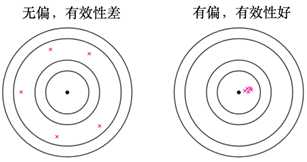左图是无偏的，结果围绕"真实值"，右侧是有偏的，结果偏向于"真实值"一侧，但是毫无疑问有偏的效果更好一些，因为更加接近真实值。

一致性

随着样本的增加，S²值将会越来越接近σ²，其实如果样本量非常大，是否有偏已经不重要，因为其实大样本情况下无论是有偏还是无偏，方差都会小到接近真实值。

附录

方差的有偏无偏估计

无偏估计：

S² = (1/n)Σ(x - μ)² ----(1)

S² = (1/n-1)Σ(x - mean(x)) -----(2)

有偏估计：

S² = (1/n)Σ(x - mean(x)) --------(3)

请注意(1)和(3)式，只是相差了一个减数，却造成了有偏和无偏，就是因为一个是固定值，一个是不定值(每次采样都不一样)。

下面我们来看一下方差无偏公式的推导过程：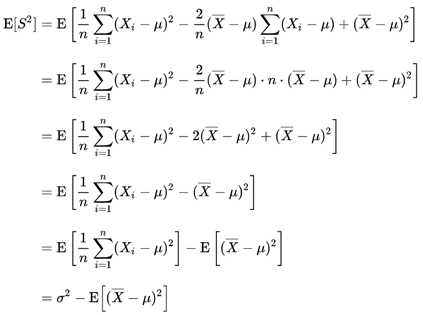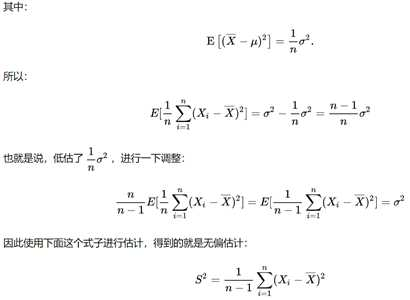参考

https://spaces.ac.cn/archives/6747数据科学网站，其中线性和非线性部分就是得子此篇文章，引发了我的对于究竟什么是分布的思考

原文：https://www.cnblogs.com/xiashiwendao/p/12213310.html

展开全文• 无偏估计在概率论和数量统计中，学习过无偏估计，最近在学习论文时候，也经常论文中提到无偏估计。虽然对无偏估计有所了解，但是还是有些问题：1)总体期望的无偏估计量是样本均值x-，总体方差的无偏估计是样本方差S^...

无偏估计

在概率论和数量统计中，学习过无偏估计，最近在学习论文时候，也经常论文中提到无偏估计。虽然对无偏估计有所了解，但是还是有些问题：

1)总体期望的无偏估计量是样本均值x-，总体方差的无偏估计是样本方差S^2,为什么样本方差需要除以n-1,而不是除以n;

2)样本在总体中是怎样的抽样过程，是放回抽样，是随机抽样，还是不放回抽样等等。

为了解决这个问题，首先来回忆一下什么叫无偏估计：

无偏估计是参数的样本估计值的期望值等于参数的真实值。估计量的数学期望等于被估计参数，则称此为无偏估计。

设A'=g(X1,X2,...,Xn)是未知参数A的一个点估计量，若A'满足

E(A')=

A

则称A'为A的无偏估计量，否则为有偏估计量。

注：无偏估计就是系统误差为零的估计。

由于公式A'=g(X1,X2,...,Xn)中的X1,X2,...,Xn一般为一次抽样的结果，没有明确是怎么抽样的一个过程，所以导致不好理解为什么A'就是A的无偏估计量，特别是很难举出实例来给与证明。经过自己的查阅资料和理解，实际上无偏估计量可以理解如下：

简单的理解，无偏估计量就是：在样本中进行n次随机的抽样，每次抽样都可以计算出一个对某一个参数的点估计量，计算n次，得到n个点估计量，然后对n个点估计量计算期望，得到的值和需要估计的总体参数相等，则称n中的任何点估计量为总体参数的无偏估计量。

能否举出一个例子呢？因为实际的应用中总体是不知道，只有样本，这能够举例子吗？是可以的，不妨设总体容量为3，样本容量为2，计算出总体方差的无偏估计为样本方差，而且样本方差是除以n-1，而不是除以n。上图为手算的两个例子，说明了总体方差的无偏估计量是样本方差，总体方差是除以n,样本方差是除以n-1。为了是上面的例子根据通用化，下面为matlab写的代码：

%

%总容量可以改变，抽样样本容量为固定2

% clc;

% clear;

% %%无偏估计验证

% %%总共容量

% M=7;

% %%样本容量

% N=2;

% %填充第一列

% %填充循环次数

% for t=1:M^(N-1)

% for i=1:M

% Sample1((i-1)*M^(N-1)+t) = i;

% end

% end

% Sample1'

% %填充第二列

% for t=1:M

% for i=1:M

% Sample2(M*(t-1)+i) = i;

% end

% end

% Sample2'

% Sample = [Sample1',Sample2']

% sLenght = length(Sample);

% for s=1:sLenght

% subSample = Sample(s,:)

% stdSample(s) = var(subSample,1);

% end

% stdSample = var(Sample');

% stdSampleE = sum(stdSample)/M^N

% Total = 1:M;

% stdTotalE = var(Total,1)

% % %总容量可以改变，抽样样本容量为固定3

% clc;

% clear;

% %%无偏估计验证

% %%总共容量

% M=7;

% %%样本容量

% N=3;

% %填充第一列

% %填充循环次数

% for t=1:M^(N-1)

% for i=1:M

% Sample1((i-1)*M^(N-1)+t) = i;

% end

% end

% Sample1'

% %填充第二列

% for t=1:M

% for i=1:M

% for j=1:M

% Sample2(M*M*(t-1)+(i-1)*M+j) = i;

% end

% end

% end

% Sample2'

% %填充第三列

% for t=1:M^2

% for i=1:M

% Sample3(M*(t-1)+i) = i;

% end

% end

% Sample = [Sample1',Sample2',Sample3']

% stdSample = var(Sample');

% stdSampleE = sum(stdSample)/M^N

% Total = 1:M;

% stdTotalE = var(Total,1)

% % %总容量可以改变，抽样样本容量为固定3

% clc;

% clear;

% %%无偏估计验证

% %%总共容量

% M=4;

% %%样本容量

% N=3;

% %填充第一列

% %填充循环次数

% for t=1:M^0

% for i=1:M

% for j=1:M^2

% Sample1(M^3*(t-1)+(i-1)*M^2+j) = i;

% end

% end

% end

% Sample1'

% %填充第二列

% for t=1:M^1

% for i=1:M

% for j=1:M^1

% Sample2(M^2*(t-1)+j+(i-1)*M) = i;

% end

% end

% end

% Sample2'

% %填充第三列

% for t=1:M^2

% for i=1:M

% for j=1:M^0

% Sample3(M^1*(t-1)+(i-1)*M^0+j) = i;

% end

% end

% end

% Sample = [Sample1',Sample2',Sample3']

% stdSample = var(Sample');

% stdSampleE = sum(stdSample)/M^N

% Total = 1:M;

% stdTotalE = var(Total,1)

clear;

%%无偏估计验证

%%总共容量

M=5;

%%样本容量

N=2;

%构造抽样的过程矩阵for

index=1:N for

t=1:M^(index-1)

for i=1:M

for j=1:M^(N-index)

Sample(M^(N-index+1)*(t-1)+(i-1)*M^(N-index)+j,index) = i;

end

end

end

end

%计算每一行的方差

varSample = var(Sample');

%计算样本方差varSampleE =

sum(varSample)/M^NTotal = 1:M;

%计算总体方差

varTotalE =

var(Total,1)

展开全文• ## 无偏估计

千次阅读 多人点赞 2019-07-04 00:43:00
0. 无偏估计简介 估计是用样本统计量（可以理解为随机抽样）来估计总体参数时的一种无偏推断。 无偏估计的要求就是：估计出来的参数的数学期望等于被估计参数的真实值。（如：是总体参数的估计量，而是被估计参数...

# 0. 无偏估计简介

估计是用样本统计量（可以理解为随机抽样）来估计总体参数时的一种无偏推断。

无偏估计的要求就是：估计出来的参数的数学期望等于被估计参数的真实值。（如：是总体参数的估计量，而是被估计参数）（无偏性是一种评价估计量优良性的准则）

无偏估计的意义：在多次重复下，估计量的平均值 ≈ 被估计参数真值

所以呢,可以看出：估计值也是一个变量，因为是随机的嘛。  真实值谁也不知道啊（因为你不可能把列出无限的实验结果来，除了可能通过数学计算得到的常见的分布）。

# 1. 无偏估计的用途

### 问题引入：

现实中想要知道全体女性的身高均值, 但是无法对所有女性测量身高，只有通过抽样一些女性，然后来估计全体女性身高的均值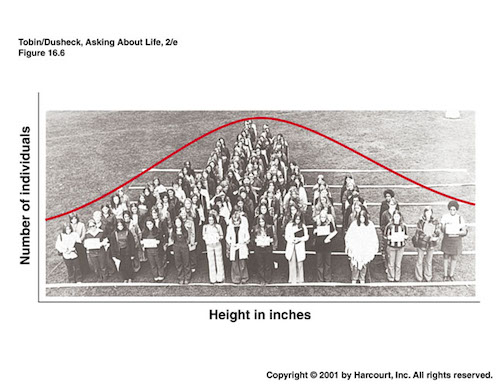# 2. 无偏估计的计算公式推导

给定一组服从一定分布的随机变量，它真实的均值和方差分别用表示，即

以女性的身高为例：

假设我们采样到的n个女性身高数据为

则样本统计的均值为

方差

此时是总体参数的无偏估计。

即判断是否成立

推导：

所以，是

### 样本方差是否是无偏估计？

即判断是否成立

推导：（为什么样本方差的分母是n-1?）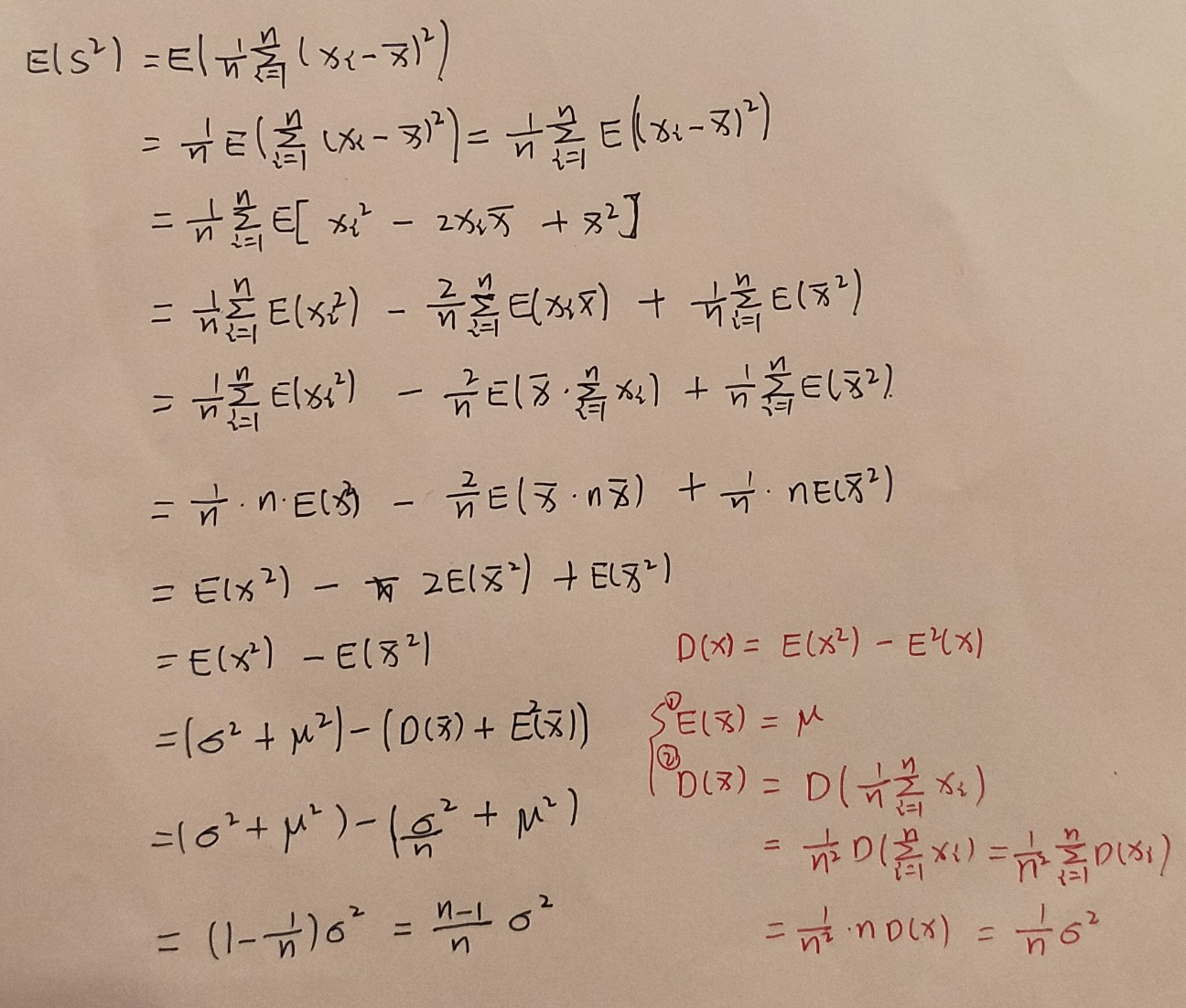所以不是的无偏估计。

，也就是低估了

所以，可以通过对做个调整，让它变为的无偏估计，即

所以的无偏估计为：

# 3. 无偏估计的直观理解

以打靶为例：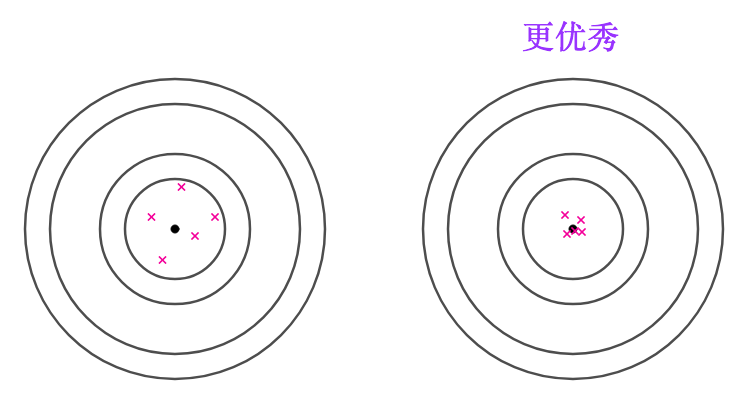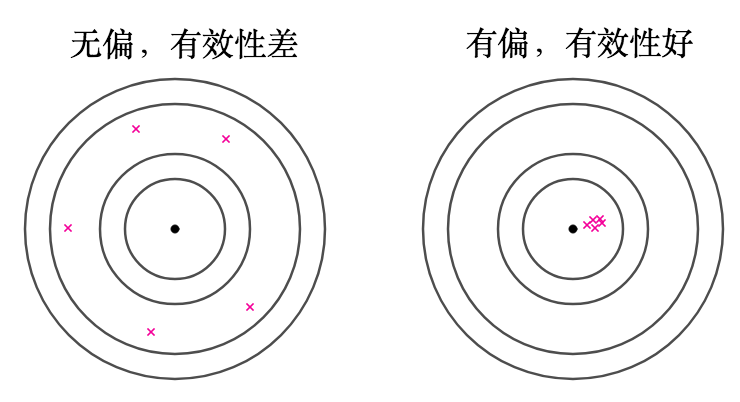左图都是无偏的，因为都在靶心周围，那么期望就是靶心

# 4. 判断估计量好坏的三种标准

判断一个估计量“好坏”，至少可以从以下三个方面来考虑：

• 无偏

• 有效

• 一致

有效性

有效性越高就说明，估计量的方差更小，估计量更靠近目标值

就像上两张图所示，可能满足无偏性，但是右边的更符合有效性

一致性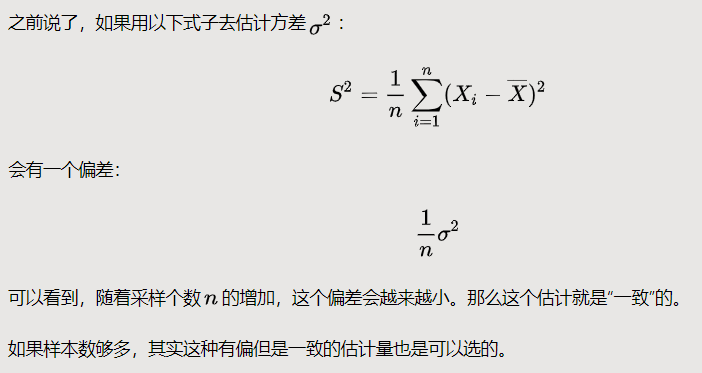实际操作中，要找到满足三个方面的量有时候并不容易，可以根据情况进行取舍。

展开全文• 讨论一般线性回归模型的影响分析问题，研究了协方差矩阵扰动和数据删除对最佳线性无偏估计的影响，给出了度量影响大小的距离测度和它的计算公式。
• 展开全部有偏估计(biased estimate)是指由样本值...无偏估计是用样本统计量来估计总体参数时的一种无偏推断。估计量的数学期望等于被估计参数的真实值，则称此估计量为被估计参数的无偏估计，即具有无偏性，是一种...
• 描述了最小方差无偏估计的基本方法和原理，附加基本例题供参考。
• 如何理解无偏估计 无偏估计：就是我认为所有样本出现的概率一样。假如有N种样本我们认为所有样本出现概率都是1/N。然后根据这个来计算数学期望。此时的数学期望就是我们平常讲的平均值。 数学期望本质就是平均值 ...
• 无偏估计和有偏估计的理解 1. 如何理解无偏估计 无偏估计：就是认为所有样本出现的概率⼀样。 数学期望本质就是平均值 假如有N种样本我们认为所有样本出现概率都是1/N。然后根据这个来计算数学期望。此时的数学期望...机器学习 深度学习
• ## 无偏估计量

千次阅读 2019-08-14 11:34:28
目录 ...5如何理解无偏估计量？ 5.1 无偏性 5.2有效性 5.3一致性 5.4 总结 参考百度百科：https://baike.baidu.com/item/%E6%97%A0%E5%81%8F%E4%BC%B0%E8%AE%A1%E9%87%8F/303853?fr=aladdin ...
• ## 理解无偏估计量

千次阅读 2020-08-25 19:23:32
理解无偏估计量 现实中常常有这样的问题，比如，想知道全体女性的身高均值 μ\muμ ，但是没有办法把每个女性都进行测量，只有抽样一些女性来对全体女性的身高进行估计. 那么根据抽样数据怎么样进行推断？什么样的...
•   无偏估计： 估计量的数学期望等于被估参数的真实值，则称此估计量为被估参数的无偏估计。(例：样本均值的期望等于总体均值，所以样本均值为无偏估计）   有偏估计：估计量的数学期望不等于被估参数的真实值，...有偏估计
• 如何理解无偏估计无偏估计有什么用？1. 如何理解无偏估计2. 无偏估计为何叫做“无偏”？它要“估计”什么？3. 为何要用无偏估计？ 1. 如何理解无偏估计 无偏估计：就是认为所有样本出现的概率⼀样。 假如有N种样本...机器学习
• 最小二乘法的无偏估计 目前我已知的最小二乘估计法有两种，第一种是基本的最小二乘法，对于白噪声，这类方法可以得到无偏估计。但对于有色噪声，这类方法只能得到有偏估计。为了解决这个问题，就导致了第二类最小...
• 有偏估计 and 无偏估计 方差分母 n-1 - 详细证明 均值 - 无偏估计 方差 - 有偏估计 方差 - 转换成无偏估计有偏估计
• 文章目录1、无偏估计量2、最小方差准则3、最小方差无偏估计的存在性4、求最小方差无偏估计量5、扩展到矢量参数 1、无偏估计量   对未知参数进行估计，得到估计量。而所谓无偏估计，是指估计量的均值，等于未知参数...
• 样本方差与无偏估计样本方差问题背景S2S^2S2的近似作用为什么使用Xˉ\bar XXˉ替代后，分母是1n\frac{1}{n}n1​？无偏估计无偏性有效性一致性小结 参考文章：如何理解无偏估计量？ by 马同学 样本方差 问题背景 首先...
• 基于Stein无偏风险估计(SURE)和阈值的线性展开式(LET),提出基于Curvelet的SURE-LET图像去噪方法。Curvelet变换实现了对于二阶可微奇异性(C2-singular-ity)分段连续目标的最优稀疏表达,同时Curvelet阈值保持了曲线......